select languageHoliday Traditionssite toolsSave this linkView your links60649 Visits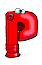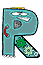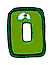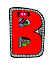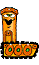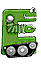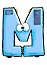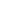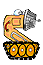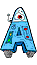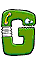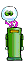Read the math problem twice. Decide what question the problem asks. Choose the correct operation Pick out the information you need for the number problem. Solve the number problem and check your answer. Read the problem again to see if your answer makes sense.   Choosing the correct operation Addition-if you read: sum, total, all together, in all, or plus   Subtraction-if you read: remainder, difference, less than, how many more, or minus   Multiplication-if you read: product, times, twice, total, or multiplied by   Division-if you read: quotient, goes into, split equally, or each